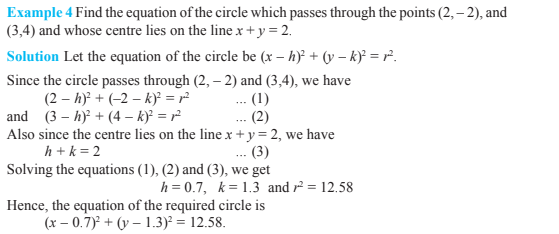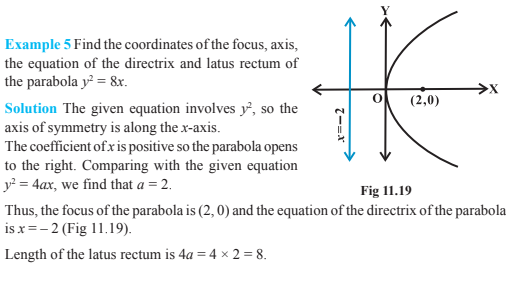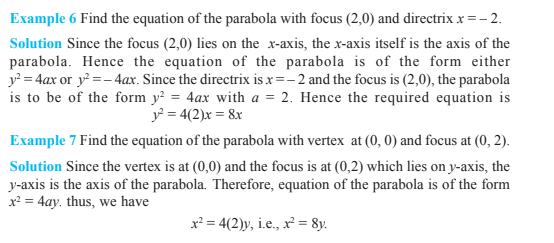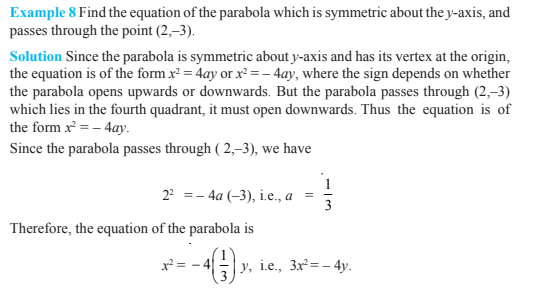# Conic Sections Class 11

In conic Sections Class 11, we will study about different kinds of curves like circles, ellipse, hyperbola and parabolas. The curves are known as conic sections or conics. Because the curves are obtained from the intersection of a plane with a double-napped right circular cone. These curves have a wide range of applications in various fields like automobile headlights, designing of antennas and telescope, reflectors etc. In conic sections, class 11 chapter 11 concepts help to understand the different sections of a cone when it is rotated.

## Conic Sections Class 11 Concepts

The topics and subtopics covered in conic sections class 11 are:

• Introduction
• Sections of a Cone
• Circle, ellipse, parabola and hyperbola
• Degenerated conic sections
• Circle
• Parabola
• Standard equations of parabola
• Latus rectum
• Ellipse
• Relationship between semi-major axis, semi-minor axis and the distance of the focus from the centre of the ellipse
• Special cases of an ellipse
• Eccentricity
• Standard equations of an ellipse
• Latus rectum
• Hyperbola
• Eccentricity
• Standard equation of Hyperbola
• Latus rectum

## Conic Sections Class 11 Notes

When a plane cuts the cone other than the vertex, we have the following situations:

(a) When β= 90°, the section is a circle

(b) When α < β < 90°, the section is an ellipse

(c) When α = β; the section is a parabola

(d) When 0 ≤ β < α; the section is a hyperbola

Where β is the angle made by the plane with the vertical axis of the cone.

Circle: Set of points in a plane equidistant from a fixed point. A circle with radius r and centre (h, k) can be represented as ( x-h)2+( y-k )2=r2

Parabola: Set of points in a plane that are equidistant from a fixed-line and point. A parabola with a > 0, focus at (a, 0), and directrix x = – a can be represented as y2=4ax

In parabola y2=4ax, the length of the latus rectum is given by 4a.

Ellipse: The sum of distances of set of points in a plane from two fixed points is constant. An ellipse with foci on the x-axis can be represented as: $\frac{x^{2}}{a^{2}}+\frac{y^{2}}{b^{2}}=1$

In ellipse $\frac{x^{2}}{a^{2}}+\frac{y^{2}}{b^{2}}=1$, the length of the latus rectum is given by $\frac{2b^{2}}{a}$.

Hyperbola: The difference of distances of set of points in a plane from two fixed points is constant. The hyperbola with foci on the x-axis can be represented as:

$\frac{x^{2}}{a^{2}}-\frac{y^{2}}{b^{2}}=1$

In a Hyperbola $\frac{x^{2}}{a^{2}}-\frac{y^{2}}{b^{2}}=1$, the length of latus rectum is given by $\frac{2b^{2}}{a}$

### Conic Sections Class 11 ExamplesStay tuned with BYJU’S – The Learning App to learn the class-wise Maths-related concepts and also refer other concepts to learn with ease.

 Also, read more class 11 concepts: Binomial Theorem Class 11 Complex Numbers Class 11 Limits and Derivatives Class 11 Linear Inequalities Class 11